## Pythagoras, a math genius? Not by Babylonian standards

Very much like today, the Old Babylonians—20th to 16th centuries BC—had the need to understand and use what is now called the Pythagoras’ (or Pythagorean) theorem. They applied it in very practical problems such as to determine how the height of a cane leaning against a wall changes with its inclination. This sounds trivial, but it was one of the most important problems studied at the time.

A remarkable Old Babylonian clay tablet, commonly referred to as Plimpton 322, was found to store combinations of three positive integers that satisfy Pythagoras’ theorem. Today we call them primitive Pythagorean triples where the term primitive implies that the side lengths share no common divisor.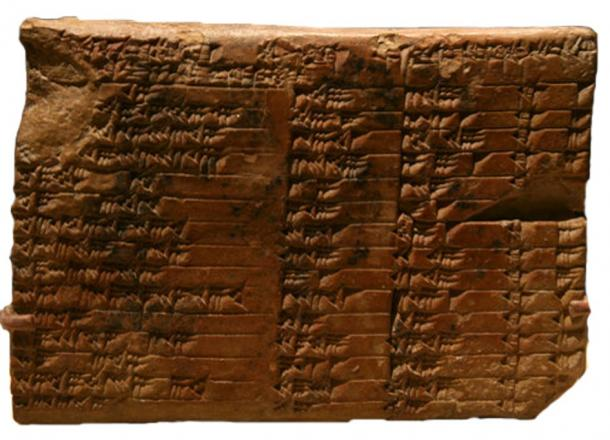Old Babylonian clay tablet (known as Plimpton 322) stores combination of primitive Pythagorean triples: (above) photo of original and (below) translated.## Why was the tablet built?

Unlike what one may imagine, the reason behind the tablet was not an interest in the number-theoretical question, but rather the need to find data for a ‘solvable’ mathematical problem. It is even believed that this tablet was a ‘teacher’s aide’ for setting up and solving problems involving right triangles. This sounds like an environment not so different from our classrooms today.

## How is this related to us?

As human beings we share the same nature as the Old Babylonians – in solving problems to live and evolve. The problems nowadays are normally more exotic and elaborate than a cane against a wall, but they share the same legacy. Right-angles are everywhere, whether it is a building, a table, a graph with axes, or the atomic structure of a crystal. While these are our contemporary challenges, we, like the Babylonians, strive to deepen our understanding of the Pythagoras’ theorem, and on the various triples that generate these useful right-angles for our everyday practical applications.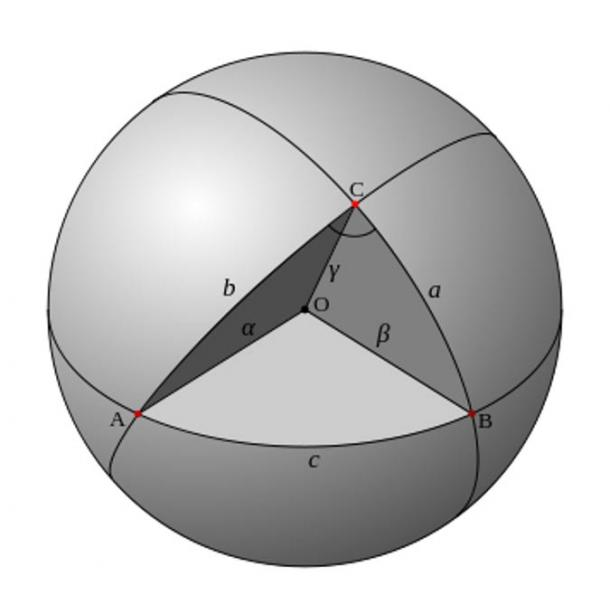Spherical trigonometry: Three right angles inside a triangle on a sphere (Public Domain)

## Were the Babylonians so different from us?

Babylonians may have used algorithms to compute side lengths of right-angled triangles into areas, and vice versa, similar to our contemporary numerical methods of analysis. These areas were farming fields, while the side lengths were canals for irrigation. Maybe the canals were structured to distribute a certain amount of water per canal.

Nowadays, in a very similar manner, computers are used to find the distribution of properties (e.g., stress, deflection, etc.) along a material (e.g., a metal beam), or even the displacement of fluids through volumes (i.e., computational fluid dynamics).

To find a solution to a problem, analytical solutions are often not available. Hence, numerical methods are employed. These consist of splitting the volume that is being analyzed (say the material of a beam, or the air in a room) into small elements (typically Platonic solids like prisms or tetrahedral). It is interesting to think that this so-called “meshing” in the engineering world, or splitting a calculation into small portions, was already applied by the old Babylonians.

Overall, one could say that the tendency to split a problem into pieces, and solve them individually to find the answer, is a human characteristic that we share with the people of Babylon. From this perspective, the Babylonians were not so different from us.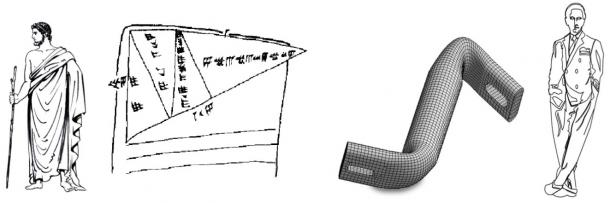Babylonian problems employed calculations similar to our contemporary numerical challenges

## Did the Babylonians know the Pythagoras’ Theorem?

Some argue that scribes in Old Babylonian period knew Pythagoras’ theorem 1,000 years before he did . The most famous tablets here – one showing a square with two diagonals, and Plimpton 322 containing a table of numerical symbols – suggests that the Babylonians knew at least some of the consequences of the theorem . Whether they derived the proof as Pythagoras did, it is unknown.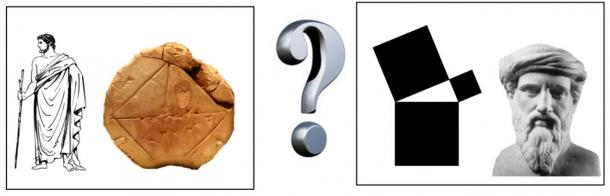A Babylonian tablet bearing a rough sketch of a square and its diagonals, and Pythagoras and his theorem

It is clear that the Pythagoras’ theorem has greatly helped humankind to evolve. It is universally applicable, but still it is exclusively binding to two dimensions. Since we live in a three dimensional world, the awareness of this gap in knowledge poses the question…

## What does the Pythagoras’ Theorem look like in three dimensions?

Until recently, this was not known. For the past five years, Luis Teia has conducted a one-man quest to shed light on this mystery. Based on his recent paper, Pythagoras triples explained via central squares published in the 2015 edition of the Australian Mathematics Senior Journal , he derived the proof. It is not philosophically farfetched to say that even the simplest things escape great minds. For example, Teia shows how to hand draw all the Pythagoras’ triples starting from the first (3,4,5) together in one outward evolving infinite spiral. This is something Pythagoras himself could have drawn some 2500 years ago, but the fact is, it eluded him and his followers, until now. Similarly, the three dimensional version of his theorem also eluded him, and is now presented.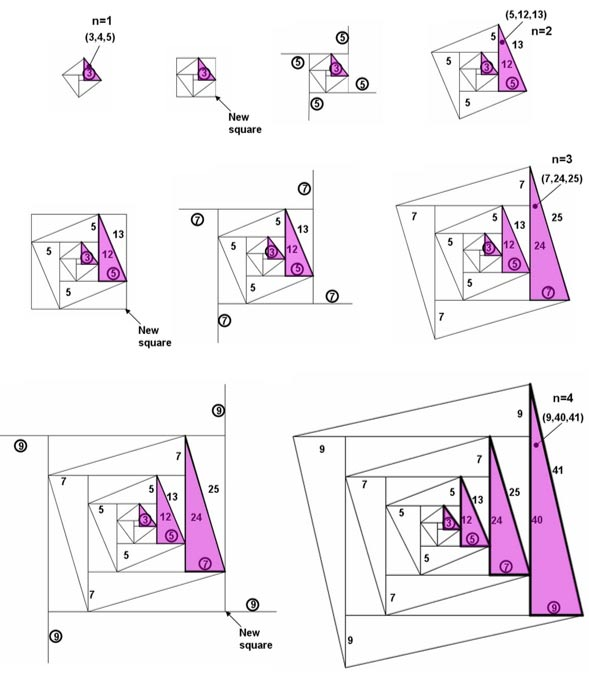Progressive construction of the Pythagoras family of triple as an outward evolving infinite spiral

## Why is the 3D Pythagorean Theorem important?

Considering the radical technological leap that Pythagoras imparted on mankind with his theorem some 2.5 millennia ago, the implications of this new 3D version can be equally significant today. Perhaps, it leads to an “upgraded” three-dimensional version of our current trigonometry. Who knows?

As for Teia’s next dream, he will be exploring the physical meaning of the three dimensional theorem (ergo the 3D “cane against the wall” problem), and the practical impact it has on contemporary science.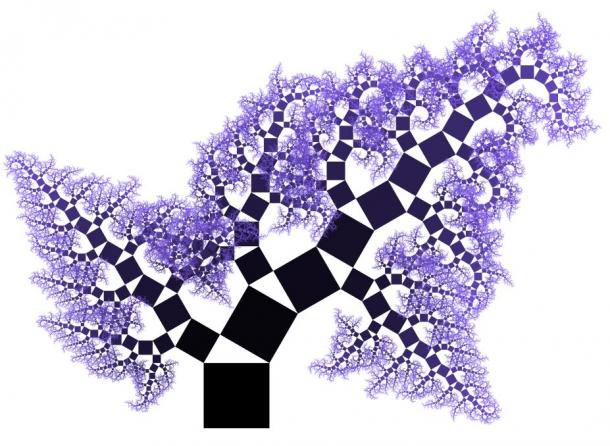A Pythagorean fractal tree

Featured image: Deriv; A brickwork lion on the ancient Babylonian Ishtar’s Gate. ( CC BY-SA 4.0 ) and Pythagorean Proof (Public Domain)

Unless otherwise noted, Images courtesy author, [Luis Teia]

By: Luis Teia

## References

Friberg, J. (1981). “Methods and traditions of Babylonian mathematics.” Historia Mathematica. Vol. 8, pp. 227-318

CNN online article. “Pythagoras, a math genius? Not by Babylonian standards” by Laura Allsop http://edition.cnn.com/2010/WORLD/meast/12/17/old.babylonian.math/ [cited 05.01.2016]

New York Times online article. “Masters of Math, From Old Babylon” by Edward Rothstein

http://www.nytimes.com/2010/11/27/arts/design/27tablets.html?_r=2 [cited 05.01.2016]

Teia, L. (2015). X3+Y3=Z3: The Proof. Book available at www.amazon.com › X3-Y3-Z3-The-Proof

Teia, L. (2015). “Pythagoras triples explained via central squares.” Australian Senior Mathematical Journal, Vol. 29, No. 1, pp. 7-15

from:

http://www.ancient-origins.net/

×